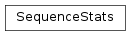# mvpa2.datasets.miscfx.SequenceStats¶class `mvpa2.datasets.miscfx.``SequenceStats`(seq, order=2)

Simple helper to provide representation of sequence statistics

WARNING: Experimental – API might change without warning! Current implementation is ugly!

Methods

 `clear`(() -> None.  Remove all items from D.) `copy`(() -> a shallow copy of D) `fromkeys`(...) v defaults to None. `get`((k[,d]) -> D[k] if k in D, ...) `has_key`((k) -> True if D has a key k, else False) `items`(() -> list of D’s (key, value) pairs, ...) `iteritems`(() -> an iterator over the (key, ...) `iterkeys`(() -> an iterator over the keys of D) `itervalues`(...) `keys`(() -> list of D’s keys) `plot`() Plot correlation coefficients `pop`((k[,d]) -> v, ...) If key is not found, d is returned if given, otherwise KeyError is raised `popitem`(() -> (k, v), ...) 2-tuple; but raise KeyError if D is empty. `setdefault`((k[,d]) -> D.get(k,d), ...) `update`(([E, ...) If E present and has a .keys() method, does: for k in E: D[k] = E[k] `values`(() -> list of D’s values) `viewitems`(...) `viewkeys`(...) `viewvalues`(...)

Initialize SequenceStats

Parameters: seq : list or ndarray Actual sequence of targets order : int Maximal order of counter-balancing check. For perfect counterbalancing all matrices should be identical

Methods

 `clear`(() -> None.  Remove all items from D.) `copy`(() -> a shallow copy of D) `fromkeys`(...) v defaults to None. `get`((k[,d]) -> D[k] if k in D, ...) `has_key`((k) -> True if D has a key k, else False) `items`(() -> list of D’s (key, value) pairs, ...) `iteritems`(() -> an iterator over the (key, ...) `iterkeys`(() -> an iterator over the keys of D) `itervalues`(...) `keys`(() -> list of D’s keys) `plot`() Plot correlation coefficients `pop`((k[,d]) -> v, ...) If key is not found, d is returned if given, otherwise KeyError is raised `popitem`(() -> (k, v), ...) 2-tuple; but raise KeyError if D is empty. `setdefault`((k[,d]) -> D.get(k,d), ...) `update`(([E, ...) If E present and has a .keys() method, does: for k in E: D[k] = E[k] `values`(() -> list of D’s values) `viewitems`(...) `viewkeys`(...) `viewvalues`(...)
`plot`()

Plot correlation coefficients